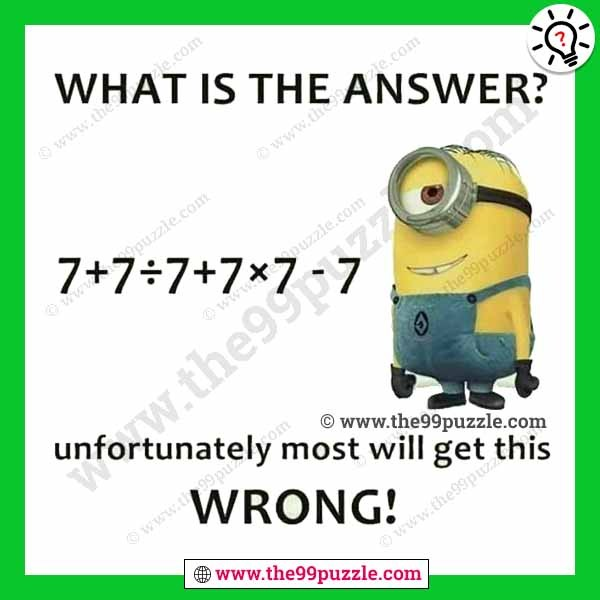# 99% of people fail to answer this problem – Puzz131

99% of people fail to answer this problem. This simple math problem can challenge your mind. If you are intelligent you can solve this. Puzzlers are very confusing to solve this math equation. If you understand the logic, find out the value of this equation?

7+7÷7+7×7−7=?###### Explanation:

In this equation simply apply the ‘BODMAS’ or ‘PEMDAS’ rule

7+7÷7+7×7−7=?

7+7÷7+(7×7)−7

7+7÷7+49−7

7+1+49−7 = 50

Math riddles or puzzles are most important in many competitive exams. We share many fun and interesting types of Brain Teaser, Math Puzzle, Number Puzzle, Word Puzzle, and Riddles. Here you also get many various types and different logic of Math riddles for teens with answers.

Here you will find many , and many more puzzles. 99% of people fail to answer this problem. We are creating various fun and interesting types of puzzles for adults and kids. Always think logically to solve easily these types of puzzles. On this website, you will play very fun games & quizzes that can improve your visual and logical thinking. Regularly we are updated new puzzles & quiz on this site. Check out daily to get new puzzles, riddles & quizzes.

### 13 thoughts on “99% of people fail to answer this problem – Puzz131”

1.2.